We have already learnt about tonic triads – how we can build a simple three-note chord based on the tonic of the key. For example, the tonic triad in the key of C major is the chord C-E-G.

In this lesson we will look at some other triads and chords which are built on other degrees of the scale – not just the tonic.

Before we do that however, let’s quickly figure out what’s the difference between a chord and a triad…

A triad is a kind of chord. All triads are chords, but not all chords are triads.

A triad is a chord with only three notes, and is built on thirds. To make a triad, we take a note, add the note a third higher, and then add another note a third higher again.

Chords

A chord contains at least two notes; it can have 3, 4, 5 or even more! In grade 4 theory you’ll only normally see chords with 3 or 4 notes. Chords with 4 notes are made by taking a simple triad and adding another note.

We can either double up one of the existing notes by writing it in a higher or lower octave:

or we can add a new note into the chord – for example another third above the highest third of the triad:

This is a chord of E major, plus D.

You won’t be tested on the second type of chord at grade 4, but you might see some of them written in the scores in the exam.

## Order of Notes

Notice that when we doubled up a note in the A minor chord, we also jumbled up the order of the other notes:

Triads are normally written with the notes as closely together as possible. We use triads when we are talking about music theory to help us to analyse harmony.

Chords are written in whichever order they sound best. We use chords in real music, not just in theory exams!

When we write down a chord, the lowest note has a special significance. The other notes can be written in any order at all – very close together, or with big gaps. (Usually in practice, there are bigger gaps between the low notes of the chord but not between the higher notes).

So, the triad of A minor, is written with A as the lowest note*, then C above, then E above that:

But the chord of A minor doubles one of the notes of the triad to make a 4-note chord. The bass note (lowest note) is A, but all the other notes can be written wherever they sound best:

(*Note – at grade 4, you only need to know about root position chords (also known as 5-3 chords). This means that the bass note is always the lowest note of the triad. In later grades you will learn that we can also change the bass note – but don’t change it just yet!!)

## Tonic, Dominant and Subdominant Triads

We always need to know what key we are in, before we start to work out chords and triads. In this part of the lesson, we’re going to look at the chords and triads in Eb major, and C minor.  Let’s start by reminding ourselves of the notes in the scale of Eb major (don’t forget to check the key signature!)

To build the tonic triad, we start on Eb, (because it’s the tonic), add a third above, and another third above:

To build the dominant triad, we start on Bb, because it’s the dominant note in Eb major. (Check the lesson on technical names of notes for more on this.) We build the triad in the same way:

To build the subdominant triad, we start on Ab, which is the subdominant note in Eb major, and do the same thing:

Triads can also be described using Roman numerals – we simply use the numeral which stands for the degree of the chord. So tonic=I (1) subdominant=IV (4) and dominant=V (5).

Let’s take a look at chords I, IV and V in C minor next. Here’s the scale of C minor (we use the harmonic minor – don’t forget to sharpen the leading note!):

The tonic is C, the subdominant is F and the dominant is G. Here are the three triads:

## How to Work out Chords and Triads

If you are given a chord or triad and are asked to name it, start by checking what key the music is in.

Next, jot down the notes you would find in chords I, IV and V (write out the scales to help you, if necessary).

Now look at the chords in the question. Match up the notes in the chord to what you’ve written down. Don’t worry about repeated notes.

That’s it! Let’s do an example together.

Name each of the numbered chords as tonic (I), subdominant (IV) or dominant (V). The key is F minor.

First we jot down the notes of the triads in F minor:

I=F-Ab-C

IV=Bb-Db-F

V=C-E natural-G

Now look at chord (1). The notes are C, C, G and E (natural) – so it’s a dominant triad.

Chord (2) has notes F, C, Ab, F, so it’s a tonic triad.

Chord (3) has notes Bb, Db, F, Db, so it’s a subdominant triad.

Don’t forget to look at:

a) the key signature,

b) all accidentals,

c) the notes in both staves if they are joined together and

d) longer notes (usually minims (half notes) or semibreves (whole notes)) which are played earlier in the bar and are still sounding as part of the chord.

Hover your mouse over the questions (tap on mobiles) to reveal the answers.

1. Identify these triads by naming the major or minor key as indicated, and describing them as tonic (I), subdominant (IV) or dominant (V)

2. Write the key signatures and triads named below.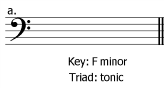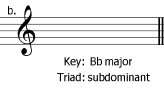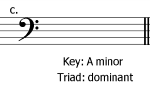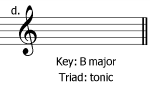3. Write the named triads as shown by the key signatures.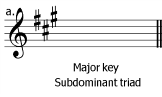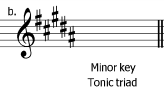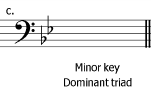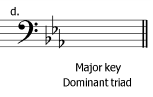4. Write the clefs and key signatures to make the triads named below.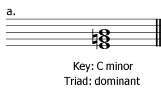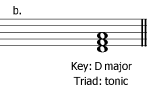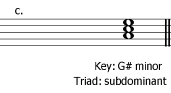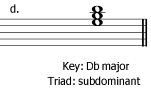5. Answer TRUE or FALSE to the following statements:

(The key of the melody is G major). Every bar from bar 1 to bar 4 contains at least one note of the dominant triad: true or false?

(The key of the melody is G minor). Every bar from bar 1 to bar 2 contains at least one note of the subdominant triad: true or false?

6. Name each of these chords as tonic (I), subdominant (IV) or dominant (V).

a. The key is D minor.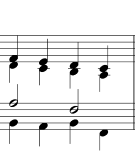b. The key is G major